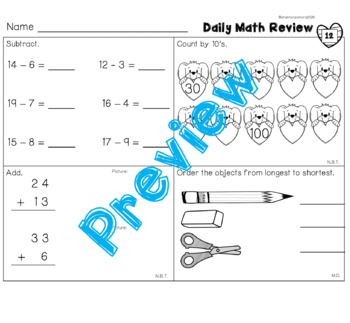# February Daily Math ReviewSubject
Resource Type
File Type

PDF

(17 MB|24 pages)
Standards
Also included in:
1. Want your students to be able to remember math concepts that you have taught throughout the year? Daily practice is key and this resource is for you. If you buy the bundle, you will have 40 weeks of spiral math review.This is a growing bundle so you will not have access to all the files right away
\$18.00
\$14.40
Save \$3.60
• Product Description
• StandardsNEW

This pack includes 4 weeks of spiral math review. It can be used as morning work, homework, or as a math warm up. This was designed to be low prep. Just print and go! It can be made into a math journal by printing the cover page, student reference sheet, and practice pages.

This is one part of a growing bundle that will provide 40 weeks of spiral math review.

The February pack includes review of the following:

•balancing equations

•story problems

•place value

•counting to 120

•measurement

•time to the hour

Determine the unknown whole number in an addition or subtraction equation relating three whole numbers. For example, determine the unknown number that makes the equation true in each of the equations 8 + ? = 11, 5 = ▯ - 3, 6 + 6 = ▯.
Understand the meaning of the equal sign, and determine if equations involving addition and subtraction are true or false. For example, which of the following equations are true and which are false? 6 = 6, 7 = 8 - 1, 5 + 2 = 2 + 5, 4 + 1 = 5 + 2.
Add and subtract within 20, demonstrating fluency for addition and subtraction within 10. Use strategies such as counting on; making ten (e.g., 8 + 6 = 8 + 2 + 4 = 10 + 4 = 14); decomposing a number leading to a ten (e.g., 13 - 4 = 13 - 3 - 1 = 10 - 1 = 9); using the relationship between addition and subtraction (e.g., knowing that 8 + 4 = 12, one knows 12 - 8 = 4); and creating equivalent but easier or known sums (e.g., adding 6 + 7 by creating the known equivalent 6 + 6 + 1 = 12 + 1 = 13).
Understand subtraction as an unknown-addend problem. For example, subtract 10 – 8 by finding the number that makes 10 when added to 8.
Apply properties of operations as strategies to add and subtract. If 8 + 3 = 11 is known, then 3 + 8 = 11 is also known. (Commutative property of addition.) To add 2 + 6 + 4, the second two numbers can be added to make a ten, so 2 + 6 + 4 = 2 + 10 = 12. (Associative property of addition.)
Total Pages
24 pages
N/A
Teaching Duration
1 month
Report this Resource to TpT
Reported resources will be reviewed by our team. Report this resource to let us know if this resource violates TpT’s content guidelines.